Maths-
General
Easy

Question

# A hostel has enough food for 125 students for 16 days. How long will the food last if 75 more students join them?Hint:

## The correct answer is: 75

### Let the number of days be represented as x and the number of students be represented as y. The number of days will decrease if the number of students are increased so we can conclude that y is in inverse relationship with x. Let’s say the proportional relationship is given asy =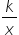……..(1)Step 1 of 2:It is given that a hostel has enough food for 125 students for 16 days i.e. x = 16 and y = 125. Putting the values in equation (1)125 =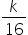k = 125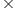16  = 2000Step 2 of 2:Now we are asked to find the number of days the food will last if 75 more students join. So, the total number of students becomes 200. Let the number of days be “d”. So, x = d and y = 200. Putting the values in equation (1)200 =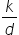Now, put the value of k = 2000d =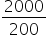d = 10 daysFinal Answer: Hence, the food will last for 10 days if 75 more students join.#### With Turito Foundation.#### Get an Expert Advice From Turito.